# A pilot points his plane due north and flies at a speed with respect to air...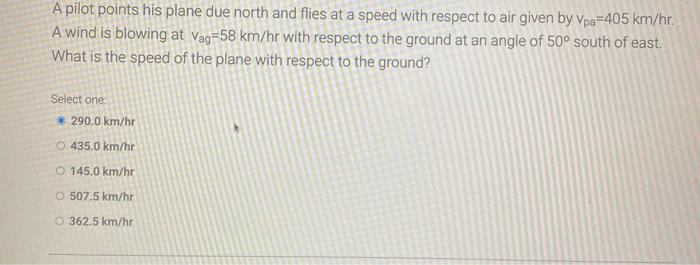A pilot points his plane due north and flies at a speed with respect to air given by Vpa=405 km/hr. A wind is blowing at Vag=58 km/hr with respect to the ground at an angle of 50° south of east. What is the speed of the plane with respect to the ground? Select one 290.0 km/hr 0 435.0 km/hr O 145.0 km/hr O 507.5 km/hr O 362.5 km/hr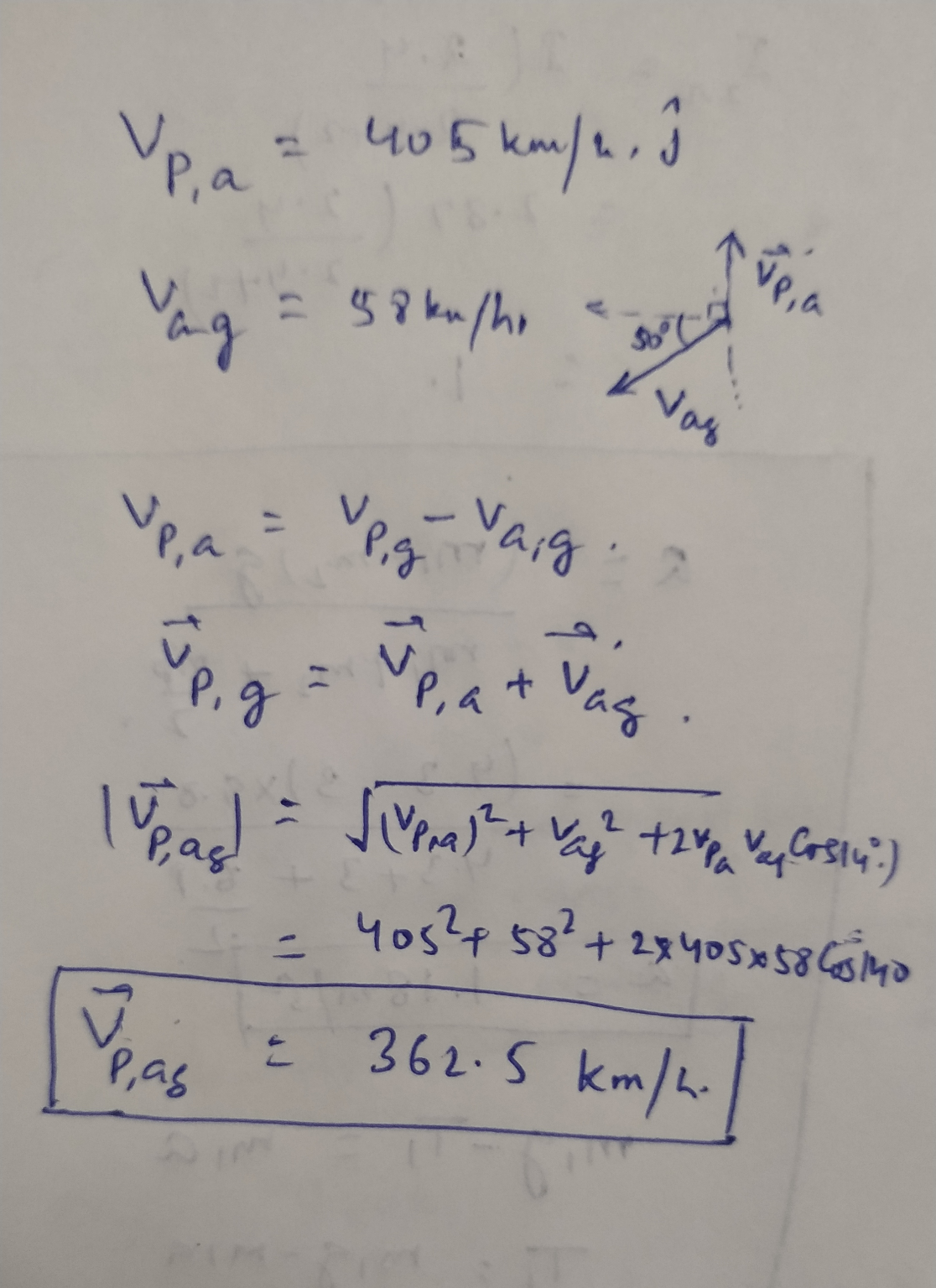##### Add Answer of: A pilot points his plane due north and flies at a speed with respect to air...
Similar Homework Help Questions
• ### A pilot points his plane due north and flies at a speed with respect to air...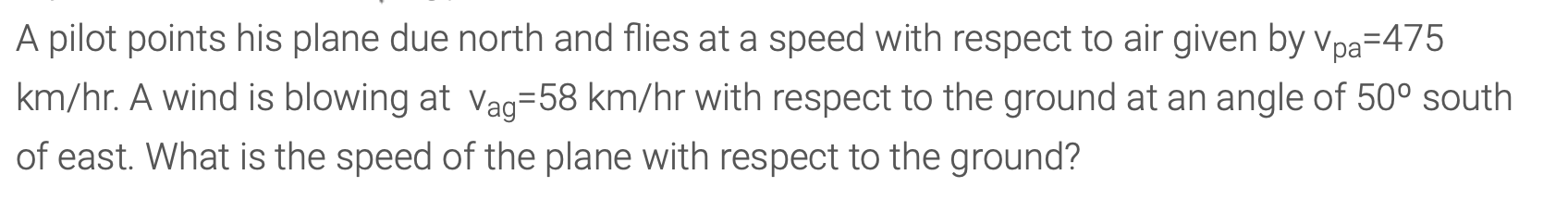A pilot points his plane due north and flies at a speed with respect to air given by vpa=475 km/hr. A wind is blowing at vag=58 km/hr with respect to the ground at an angle of 50º south of east. What is the speed of the plane with respect to the ground? A pilot points his plane due north and flies at a speed with respect to air given by Vpa=475 km/hr. A wind is blowing at Vag=58 km/hr with...

• ### A pilot points his plane due north and flies at a speed with respect to air...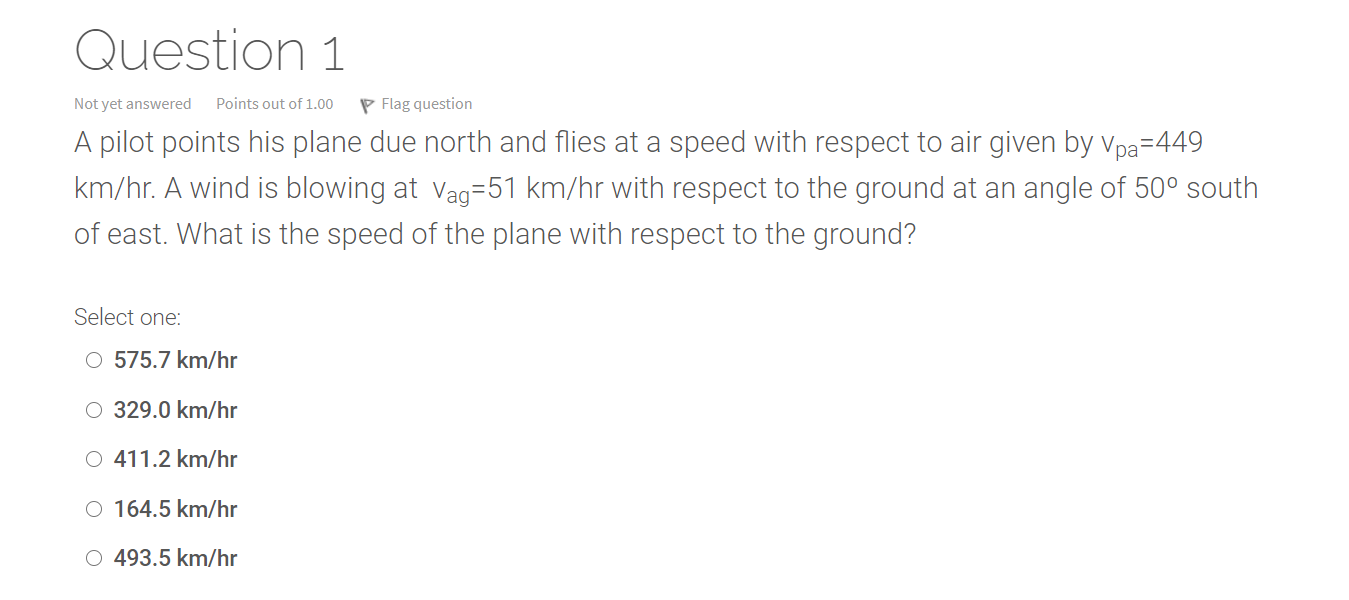A pilot points his plane due north and flies at a speed with respect to air given by vpa=449 km/hr. A wind is blowing at vag=51 km/hr with respect to the ground at an angle of 50º south of east. What is the speed of the plane with respect to the ground? Question 1 Not yet answered Points out of 1.00 Flag question A pilot points his plane due north and flies at a speed with respect to air given...

• ### A pilot points his plane due north and flies at a speed with respect to air...

A pilot points his plane due north and flies at a speed with respect to air given by vpa=475 km/hr. A wind is blowing at vag=58 km/hr with respect to the ground at an angle of 50º south of east. What is the speed of the plane with respect to the ground?

• ### A pilot points his plane due north and flies at a speed with respect to air...

A pilot points his plane due north and flies at a speed with respect to air given by vpa=406 km/hr. A wind is blowing at vag=43 km/hr with respect to the ground at an angle of 50º south of east. What is the speed of the plane with respect to the ground?

• ### A pilot points his plane due north and flies at a speed with respect to air...

A pilot points his plane due north and flies at a speed with respect to air given by vpa=462 km/hr. A wind is blowing at vag=46 km/hr with respect to the ground at an angle of 50º south of east. What is the speed of the plane with respect to the ground?

• ### A pilot points his plane due north and flies at a speed with respect to air...

A pilot points his plane due north and flies at a speed with respect to air given by vpa=475 km/hr. A wind is blowing at vag=58 km/hr with respect to the ground at an angle of 50º south of east. What is the speed of the plane with respect to the ground? Select one: a) 345.7 km/hr b) 172.9 km/hr c) 605.1 km/hr d) 518.6 km/hr e) 432.2 km/hr

• ### 1. A pilot points his plane due north and flies at a speed with respect to...

1. A pilot points his plane due north and flies at a speed with respect to air given by vpa=425 km/hr. A wind is blowing at vag=41 km/hr with respect to the ground at an angle of 50º south of east. What is the speed of the plane with respect to the ground?

• ### a pilot points his plane due north and flies at a soeed with respect to air...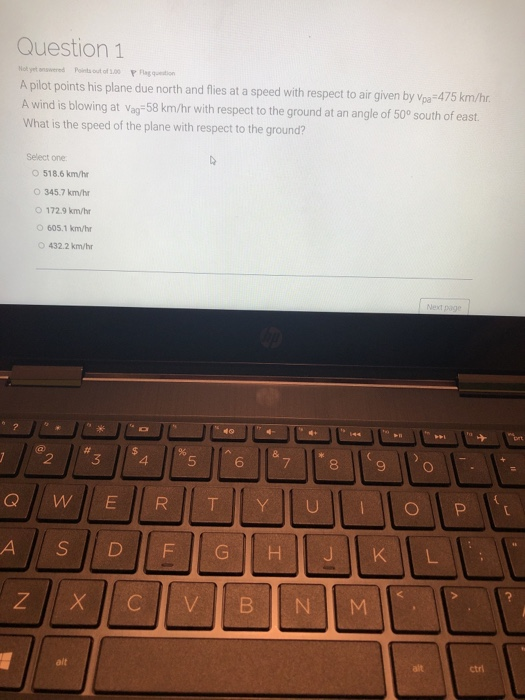a pilot points his plane due north and flies at a soeed with respect to air given by Vpa=475km/hr. A wind is blowing at Vag= 58km/hr with respect to the ground at an angle of 50degrees south of east. What is the speed of the plane with respect to the ground? Question 1 Hoyerswered Points out of Praction A pilot points his plane due north and flies at a speed with respect to air given by Vpa=475 km/hr. A wind...

• ### A pilot points his plane due north and flies at a speed with respect to air...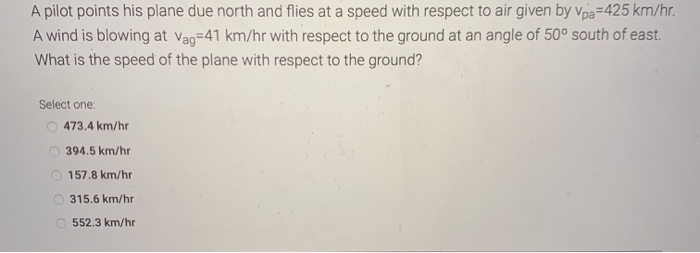A pilot points his plane due north and flies at a speed with respect to air given by Vpa=425 km/hr. A wind is blowing at Vag=41 km/hr with respect to the ground at an angle of 50° south of east. What is the speed of the plane with respect to the ground? Select one: 473.4 km/hr 394.5 km/hr 157.8 km/hr 315.6 km/hr 552.3 km/hr

• ### A pilot points his plane due north and flies at a speed with respect to air...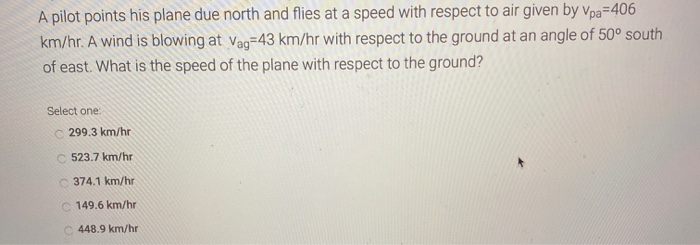A pilot points his plane due north and flies at a speed with respect to air given by Vpa=406 km/hr. A wind is blowing at Vag=43 km/hr with respect to the ground at an angle of 50° south of east. What is the speed of the plane with respect to the ground? Select one: C 299.3 km/hr C523.7 km/hr 374.1 km/hr 149.6 km/hr 448.9 km/hr The figure below shows two masses m1=4.5 kg and m2=2.0 kg connected to separate ropes...

Free Homework App